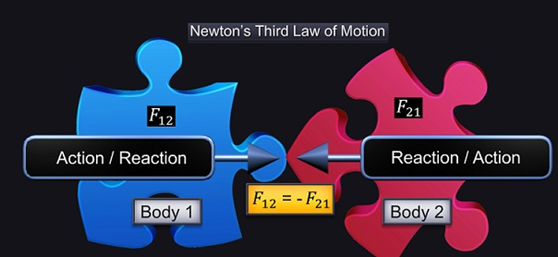Notes On Newton's Third Law of Motion - CBSE Class 11 Physics
Newton’s Third Law of Motion states that: “To every action there is always an equal and opposite reaction.” Action and reaction are forces that come into existence at the same instant. Action and reaction forces act on different bodies. Mathematically, Newton’s Third Law of Motion can also be written as shown.Here, F12 is the force applied by body 1 on body 2 and F21 is the force applied by body 2 on body 1. The minus sign indicates the opposite direction.   Forces always occur in pairs. Force on a body A by body B is equal and opposite to the force on body B by body A. If two bodies A and B exist within a system, then force of A on B and force of B and A become internal forces of the system and they cancel each other.

#### Summary

Newton’s Third Law of Motion states that: “To every action there is always an equal and opposite reaction.” Action and reaction are forces that come into existence at the same instant. Action and reaction forces act on different bodies. Mathematically, Newton’s Third Law of Motion can also be written as shown.Here, F12 is the force applied by body 1 on body 2 and F21 is the force applied by body 2 on body 1. The minus sign indicates the opposite direction.   Forces always occur in pairs. Force on a body A by body B is equal and opposite to the force on body B by body A. If two bodies A and B exist within a system, then force of A on B and force of B and A become internal forces of the system and they cancel each other.

Next